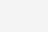# 两大数据类型

• 基本数据类型：number\string\boolean\null\undefined\symbol\bigint
• 引用数据类型：object\function
• symbol
``````//Symbol.for()
Symbol.for() 类似单例模式，首先会在全局搜索被登记的 Symbol 中是否有该字符串参数作为名称的 Symbol值，

let yellow = Symbol("Yellow");
let yellow1 = Symbol.for("Yellow");
yellow === yellow1; // false
let yellow2 = Symbol.for("Yellow");
yellow1 === yellow2; // true
//Symbol.keyFor()
Symbol.keyFor() 返回一个已登记的 Symbol 类型值的 key ，用来检测该字符串参数作为名称的 Symbol 值是否已被登记。
Symbol.keyFor()获取的只能是symbol.for获取的值
let yellow1 = Symbol.for("Yellow");
Symbol.keyFor(yellow1); // "Yellow"
``````

# 类型判断方法

``````typeof

instanceof
### instanceof重写判断基本数据类型
class PrimitiveNumber {
static [Symbol.hasInstance](x) {
return typeof x === 'number'
}
}
console.log(111 instanceof PrimitiveNumber) // true
//手动实现一下instanceof
function myInstanceof(left, right) {
//基本数据类型直接返回false
if(typeof left !== 'object' || left === null) return false;
//getProtypeOf是Object对象自带的一个方法，能够拿到参数的原型对象
let proto = Object.getPrototypeOf(left);
while(true) {
//查找到尽头，还没找到
if(proto == null) return false;
//找到相同的原型对象
if(proto == right.prototype) return true;
proto = Object.getPrototypeof(proto);
}
}
//测试:
console.log(myInstanceof("111", String)); //false
console.log(myInstanceof(new String("111"), String));//true

constructor

Oject.prototype.toString.call
``````
• typeof出来的一定是字符串
``````let a=typeof typeof typeof [a,b] =>"string "
type Infinity='number'
type NaN='number'
``````
• null不是对象
``````虽然 typeof null 会输出 object，但是这只是 JS 存在的一个悠久Bug。

000 开头代表是对象然而 null 表示为全零，所以将它错误的判断为 object
``````
• 如何让if(a == 1 && a == 2)条件成立？
``````var a = {
value: 0,
valueOf: function() {
this.value++;
return this.value;
}
};
console.log(a == 1 && a == 2);//true
``````
• 特殊类型NaN
``````NaN!=NaN NaN和谁都不相等，监测用isNaN()
//isNaN 使用的时候先隐形转换为数字
``````
• parseFloat/parseInt
``````//parseFloat 寻找有效字符，如果第一个不是就不找了，否则找到知道不是有效字符的字符
//parseInt不能识别小数点
parseFloat("12.5px") =>12.5

let res=parseFloat('left:200px') =>NaN
if(res===200){
}else if(res===NaN){
}else if(typeof res==='number'){
}else {
}
``````

parseInt(string, radix) `string：要解析的值，非字符串内部会先转化为字符串处理，开头结尾的空格会被忽略` `radix：可选参数，数字基数，可以理解为进制，范围为2~36`

``````radix记住0， undefined， null三种特殊的情况，

``````
• parseFloat/parseInt和Number
``````//Number是底层的东西：
true 1
false 0
null 0
undefined NaN
"" 0
[] 0
//字符串必须保证都是有效数字，否则使用number转换都是NaN

parseInt("") =》NaN
Number("") => 0
isNaN("") =>false
parseInt(null) =>NaN
Number(null) =>0
isNaN(null) => FALSE
parseInt("12px") =>12
Number("12px") =>NaN
isNaN("12px") =>true
parseFloat("1.6px")+parseInt("1.2px")+typeof parseInt(null) =>1.6+1+'number'=>'2.6number' isNaN(Number(!!Number(parseInt("0.8")))) =>false
typeof !parseInt(null)+!isNaN(null) =>'booleantrue'
[]==true =>false  //都需要转换为数字 0==1
window.isNaN()  =>true
``````
• 布尔值
``````//只有0/NaN/null/undefined/'' 转为为布尔值为false 其余都是true
0==null false
[]==false
![]==false
[] == ![] //都转换未number比较，左边为0 右边线转换为布尔值![]为false,转为number即0，所以相等
``````
• 类型转换
• 对象=字符串 转换为字符串,遇到对象要把对象转换为字符串
• null==undefined 但是和其他值都不相等
• 剩下两边不同都是转换为数字
``````//字符串对比字符串，则都转换为unicode进行比较

{}.tostring() =>[object Object]
let result=10+false+undefined+[]+'Tencent'+null+true+{}
console.log(result)
=>
10+0=10
10+undefined NaN
NaN+[] ‘NaN’
'NaNTecnent'
.....
'NaNTencentnulltrue[object Object]'
``````
• 运算
``````//一旦出现字符串或者对象运算，那就一定是字符串拼接
NaN+'tECENT'='NaNtECENT'
//
0.1+0.2为什么不等于0.3？
0.1和0.2在转换成二进制后会无限循环，由于标准位数的限制后面多余的位数会被截掉，

//Object.is和===的区别
Object在严格等于的基础上修复了一些特殊情况下的失误，具体来说就是+0和-0，NaN和NaN。 源码如下：
function is(x, y) {
if (x === y) {
//运行到1/x === 1/y的时候x和y都为0，但是1/+0 = +Infinity， 1/-0 = -Infinity, 是不一样的
return x !== 0 || y !== 0 || 1 / x === 1 / y;
} else {
//NaN===NaN是false,这是不对的，我们在这里做一个拦截，x !== x，那么一定是 NaN, y 同理
//两个都是NaN的时候返回true
return x !== x && y !== y;
}
``````
• 大括号{}
``````//{}大括号在js中被认为可以是对象、代码块、等
{}+0=0   //大括号被认为是代码快所以直接加0为0
//+加号都是转换为数字运算
{}+0？1:0 =>0 //{}代码快
({}+0) =>"[object Object]0" //{}运算
({})+0 =>"[object Object]0" //{}运算
0+{} =》"0[object Object]" //{}运算
(function AAA(){}+0)  =>"function AAA(){}0" 类似大括号
``````

• 1.在没有使用小括号处理优先级的情况下 不认为是数学运算，加小括号才算
• 2.出现在运算符的后面 认为是数学运算
``````特殊：{}+0+{} =>“[object Object]0[object Object]”
``````
• 伪数组转换为数组
``````Array.prototype.slice.call(OBJ)
``````

# 对象转原始类型是根据什么流程运行的

• 如果Symbol.toPrimitive()方法，优先调用再返回
• 调用valueOf()，如果转换为原始类型，则返回
• 调用toString()，如果转换为原始类型，则返回
• 如果都没有返回原始类型，会报错
``````var obj = {
value: 3,
valueOf() {
return 4;
},
toString() {
return '5'
},
[Symbol.toPrimitive]() {
return 6
}
}
console.log(obj + 1); // 输出7
``````

# 堆栈知识

1. 概念
``````GO（global object） 全局对象 堆内存
EC (Execution Context) 执行上下文
VO (Varibale Object) 变量对象
VOG(Varibale Object Global) 全局变量对象
Ao(Activation Object) 活动变量对象 VO的一个分支
``````
• 基本类型值直接存栈内部就行，引用类型值单独开辟新堆存储。
• 为什么叫引用类型，变量使用的知识堆中引用类型的地址，所以叫引用类型， 而基本类型是变量直接使用在栈中的基本类型值
• 特殊：闭包变量是存在堆内存中的
``````var a=12 var b=a b=13 console.log(a) =>12

var a={a:12} var b=a b['n']=13 console.log(a.n) =>13

var a={n:12} var b=a b={n:13} console.log(a.b) =>12

var obj={ name:'12', fn:(function(x){ return x+10; })(obj.name) =》报错不存在name，而不是报obj不存在，因为存在变量提升 } console.log(obj.fn) //报错

var a={},b={m:'1'},c={n:'2'} a[b]='11' a[c]='22' console.log(a[b]) =>22

var a={n:1} var b=a a.x=a={n:2} //a.x赋值操作高于a本身赋值 console.log(a.x) console.log(b)

``````
• 为什么不用栈来存储复杂数据类型 对于系统栈来说，它的功能除了保存变量之外，还有创建并切换函数执行上下文的功能
``````function f(a) {
console.log(a);
}

function func(a) {
f(a);
}

func(1);
``````

1. 调用func, 将 func 函数的上下文压栈，ESP指向栈顶。
1. 执行func，又调用f函数，将 f 函数的上下文压栈，ESP 指针上移。
1. 执行完 f 函数，将ESP 下移，f函数对应的栈顶空间被回收。
1. 执行完 func，ESP 下移，func对应的空间被回收(0)

### 相关推荐

• #### 分布式事务，原理简单，写起来全是坑！[通俗易懂]

分布式事务，原理简单，写起来全是坑！[通俗易懂]分布式事务，我们已经给小伙伴介绍了整体内容： 一文搞明白分布式事务解决方案！真的 so easy！ AT 模式的实现： 手把手带领小伙伴们写一个分布式事务案例！ AT 模式在多数据源中的应用： Spr

• #### mysqlinnodb事务隔离级别_数据库默认的事务隔离级别

mysqlinnodb事务隔离级别_数据库默认的事务隔离级别MySQL 事务隔离级别是为了解决并发事务互相干扰的问题的，MySQL 事务隔离级别总共有以下 4 种：

• #### 一文彻底掌握MySQL事务(四大特性，四种隔离级别，七种传播行为)[通俗易懂]

一文彻底掌握MySQL事务(四大特性，四种隔离级别，七种传播行为)[通俗易懂]小知识，大挑战！本文正在参与“程序员必备小知识”创作活动。事务四大特性原子性（Atomicity）原子性指整个事务是不可分割的工作单位。在事务中

• #### 计算机网络-应用层-DHCP协议

计算机网络-应用层-DHCP协议什么是DHCP协议 动态主机配置协议DHCP（Dynamic Host Configuration Protocol）是一种网络管理协议，用于集中对用户IP地址进行动态管理和配置。 DHCP于1993

• #### 云计算核心技术剖析（云计算使用的主要技术）

云计算核心技术剖析（云计算使用的主要技术）

• #### HTML学习笔记（10）表单元素的特点、代码和显示效果

HTML学习笔记（10）表单元素的特点、代码和显示效果这一章学习表单元素。表单元素是HTML中最复杂的内容，很多名词容易混淆。希望通过系统地学习比较，掌握好它们。学无止境，乐在其中。点击关注，一起进

• #### 堆排序算法（堆排序和快速排序对比）

堆排序算法（堆排序和快速排序对比）

• #### 【C 语言】数组作为参数退化为指针问题

【C 语言】数组作为参数退化为指针问题文章目录 一、问题描述 二、从编译器角度分析该问题 三、数组作为参数的推荐方案 一、问题描述 将 数组 作为 函数参数 , 传递时会 退化为指针 ; 数组的首地址 , 变为指针地址 , 函数中无法判定